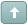Error

 Previous Topic Next Topic
 gorachand #1 Posted : Monday, December 30, 2013 10:29:49 PM(UTC)Rank: Advanced MemberGroups: Registered, Registered UsersJoined: 2/19/2012(UTC)Posts: 78Thanks: 1 times A De Mark Count Down is completed when:-1. The low of TD Buy Countdown bar thirteen must be less than, or equal to, theclose of TD Buy Countdown bar eight, and2. The close of TD Buy Countdown bar thirteen must be less than, or equal to, thelow two bars earlierI first plot the following indicatorsTD - SetUp-buyTD1:=If(CLOSE=Ref(C,-13),1,0); {>= may be changed in >}TD4:=If(TD2=1 AND TD3=1,1,0); TD5:=If(HIGH>=Ref(LLV(LOW,5),-3),1,0); D8:=If(Ref(TD4,-1)=1 AND TD5=1,1,0); D9:=If(TD4=1 AND TD5=1 AND Ref(D8,-1)<>1,1,0); D10:=If(Ref(TD4,-1)=1 AND TD5=1 AND Ref(D8,-2)<>1 AND Ref(D9,-1)<>1,1,0); D11:=If(Ref(TD4,-2)=1 AND TD5=1 AND Ref(D8,-3)<>1 AND Ref(D9,-2)<>1 AND Ref(D10,-1)<>1,1,0); D12:=If(Ref(TD4,-3)=1 AND TD5=1 AND Ref(D8,-4)<>1 AND Ref(D9,-3)<>1 AND Ref(D10,-2)<>1 AND Ref(D11,-1)<>1,1,0); D13:=If(Ref(TD4,-4)=1 AND TD5=1 AND Ref(D8,-5)<>1 AND Ref(D9,-4)<>1 AND Ref(D10,-3)<>1 AND Ref(D11,-2)<>1 AND Ref(D12,-1)<>1,1,0); D14:=If(Ref(TD4,-5)=1 AND TD5=1 AND Ref(D8,-6)<>1 AND Ref(D9,-5)<>1 AND Ref(D10,-4)<>1 AND Ref(D11,-3)<>1 AND Ref(D12,-2)<>1 AND Ref(D13,-1)<>1,1,0); D15:=If(Ref(TD4,-6)=1 AND TD5=1 AND Ref(D8,-7)<>1 AND Ref(D9,-6)<>1 AND Ref(D10,-5)<>1 AND Ref(D11,-4)<>1 AND Ref(D12,-3)<>1 AND Ref(D13,-2)<>1 AND Ref(D14,-1)<>1,1,0); D16:=If(Ref(TD4,-7)=1 AND TD5=1 AND Ref(D8,-8)<>1 AND Ref(D9,-7)<>1 AND Ref(D10,-6)<>1 AND Ref(D11,-5)<>1 AND Ref(D12,-4)<>1 AND Ref(D13,-3)<>1 AND Ref(D14,-2)<>1 AND Ref(D15,-1)<>1,1,0); D17:=If(Ref(TD4,-8)=1 AND TD5=1 AND Ref(D8,-9)<>1 AND Ref(D9,-8)<>1 AND Ref(D10,-7)<>1 AND Ref(D11,-6)<>1 AND Ref(D12,-5)<>1 AND Ref(D13,-4)<>1 AND Ref(D14,-3)<>1 AND Ref(D15,-2)<>1 AND Ref(D16,-1)<>1,1,0); SetUp:=D8+D9+D10+D11+D12+D13+D14+D15+D16+D17; SetUpTD Count Down Buy ACum(If(CLOSE 14Fml( "TD Count Down Buy A" ) =9 AND Ref( Fml( "TD Count Down Buy A" ) ,-1 )<> 9Then I plot the exploration formulaA:=Fml( "TD Count Down Buy A" ) =9 AND Ref( Fml( "TD Count Down Buy A" ) ,-1 )<> 9;B:=Fml( "TD Count Down Buy A" ) =14 AND Ref( Fml( "TD Count Down Buy A" ) ,-1 )<> 14;B AND L<=A AND C<= Ref(L,-2)I plug this into filter but the exploration gives a 100% rejection. Where am I going wrong?Can anybody help?If Bar 13Fml( "TD Count Down Buy A" ) =14 AND Ref( Fml( "TD Count Down Buy A" ) ,-1 )<> 14can be modified in the expert code including the conditions1. The low of TD Buy Countdown bar thirteen must be less than, or equal to, theclose of TD Buy Countdown bar eight, and2. The close of TD Buy Countdown bar thirteen must be less than, or equal to, thelow two bars earlier----that would definitely solve the problem.Regards,Dr.ChatterjeeWanna join the discussion?! Login to your Discussions forum accountor Register a new forum account.Users browsing this topic
Forum Jump
You cannot post new topics in this forum.
You cannot reply to topics in this forum.
You cannot delete your posts in this forum.
You cannot edit your posts in this forum.
You cannot create polls in this forum.
You cannot vote in polls in this forum.